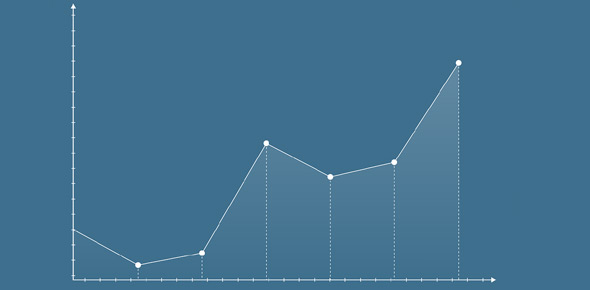# What Type Of Graph Should You Use?

Approved & Edited by ProProfs Editorial Team
At ProProfs Quizzes, our dedicated in-house team of experts takes pride in their work. With a sharp eye for detail, they meticulously review each quiz. This ensures that every quiz, taken by over 100 million users, meets our standards of accuracy, clarity, and engagement.
| Written by AlisonWerstuk
A
AlisonWerstuk
Community Contributor
Quizzes Created: 1 | Total Attempts: 754
Questions: 6 | Attempts: 757SettingsThis is the quiz for APC students studying graphing and Statistics. Put a checkmark in the box for the type of graph you would use for the data given.

• 1.

### Colours of smarties:

• A.

Column graph

• B.

Double column graph

• C.

Histogram

A. Column graph
Explanation
A column graph is the most suitable representation for the colours of smarties because it allows for easy comparison between different categories (in this case, different colours of smarties). Each colour can be represented by a separate column, and the height of the column can represent the frequency or quantity of that particular colour. This visual representation helps in quickly understanding the distribution of colours and identifying any patterns or trends. A double column graph or histogram may not be as appropriate as they are typically used for different types of data analysis.

Rate this question:

• 2.

### Heights of students in cm:

• A.

Column graph

• B.

Double column graph

• C.

Histogram

C. Histogram
Explanation
A histogram is the most appropriate choice for representing the heights of students in cm because it is a graphical representation that displays the distribution of a continuous variable. In this case, the heights of students can be categorized into different bins or intervals, and the frequency or number of students falling within each interval can be represented by the height of the bars. This allows us to easily visualize the distribution of heights and identify any patterns or trends. A column graph or double column graph may not be suitable as they are typically used for comparing discrete categories or groups, rather than representing a continuous variable like height.

Rate this question:

• 3.

### How many songs you have on iTunes:

• A.

Column graph

• B.

Double column graph

• C.

Histogram

C. Histogram
Explanation
A histogram is the most appropriate type of graph to represent the number of songs on iTunes because it allows for the display of the frequency distribution of the data. In this case, the histogram would have the number of songs on the x-axis and the frequency (or count) of each number of songs on the y-axis. This would provide a visual representation of how many songs are present in different ranges or categories, allowing for easy comparison and analysis of the data.

Rate this question:

• 4.

### How many siblings students have:

• A.

Column graph

• B.

Double column graph

• C.

Histogram

A. Column graph
Explanation
A column graph is a type of chart that uses vertical bars to represent data. Each bar represents a category, in this case, the number of siblings students have. The height of the bar corresponds to the frequency or count of students in each category. This type of graph is suitable for comparing the number of siblings among different groups or categories. It provides a clear visual representation of the data and allows for easy comparison between categories.

Rate this question:

• 5.

### The favourite subjects of boys and girls separately:

• A.

Column graph

• B.

Double column graph

• C.

Histogram

B. Double column graph
Explanation
A double column graph is the most suitable option to represent the favorite subjects of boys and girls separately. This type of graph allows for a clear comparison between two different groups, in this case, boys and girls. The double column graph displays two sets of columns side by side, making it easy to compare the data for each group. This type of graph is commonly used to compare two related variables and is ideal for representing categorical data, such as favorite subjects.

Rate this question:

• 6.

### The cost of five items in Australia and the cost of the same five items in the UK:

• A.

Column graph

• B.

Double column graph

• C.

HistogramBack to top